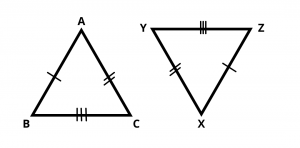# What is SSS (Side Side Side) Congruence Rule ?

## Definition :

If three sides of one triangle are equal to the three sides of another triangle, then the two triangles are congruent. This is Known as SSS Congruency.For given two triangles,  AB = XZ , AC = XY and BC = YZ, then using SSS rule, $$\Delta$$ ABC ≅ $$\Delta$$ XYZ.

### Similar Questions

What is SAS (Side Angle Side) Congruence Rule ?

What is RHS (Right angle Hypotenuse Side) Congruence Rule ?

What is ASA (Angle Side Angle) Congruence Rule ?

What is AAS (Angle Angle Side) Congruence Rule ?